## q-Dimension(1)

where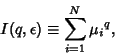(2)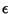is the box size, andis the Natural Measure. If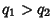, then(3)

The Capacity Dimension (a.k.a. Box Counting Dimension) is given by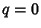,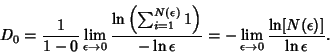(4)

If alls are equal, then the Capacity Dimension is obtained for any. The Information Dimension is defined by(5)

But(6)

so use L'Hospital's Rule(7)

Therefore,(8)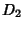is called the Correlation Dimension. The-dimensions satisfy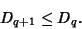(9)